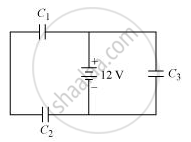Advertisement Remove all ads

# Three Identical Capacitors C1, C2 and C3 of Capacitance 6 μF Each Are Connected to a 12 V Battery as Shown.Find(I) Charge on Each Capacitor(Ii) Equivalent Capacitance of the Network(Iii) Energy Stored - Physics

Advertisement Remove all ads
Advertisement Remove all ads
Advertisement Remove all ads

Three identical capacitors C1, C2 and C3 of capacitance 6 μF each are connected to a 12 V battery as shown.Find

(i) charge on each capacitor

(ii) equivalent capacitance of the network

(iii) energy stored in the network of capacitors

Advertisement Remove all ads

#### Solution

The 12 V battery is in parallel with C1, C2, and C3. C1 and C2are in series with each other while Cis in parallel with the combination formed by C1and C2.

Total voltage drop across C3 = 12 V

q3 = CV

Where, q = Charge on the capacitor

C1, C2, C3 = 6 μF (Given in the question)

q3 = 6 × 12 = 72 μC

Voltage drop across C1 and C2 combined will be 12 V.

Let the voltage drop at C1 = V1

Let the voltage drop at C2 = V2

Then,

V = V1 + V2

V_1 = q_1/C

V_2 = q_2/C

q_1/6+q_2/6 = 12

As both the capacitors are in series,

q_1 =q_2 =q

Then,

q{1/6 +1/6} =12

q xx 1/3 =12

Or,

q = 36 micro coulombs

Thus, charge on each of C1 and C2 is 36 coulombs.

Concept: Capacitors and Capacitance
Is there an error in this question or solution?

#### Video TutorialsVIEW ALL 

Advertisement Remove all ads
Share
Notifications

View all notifications

Forgot password?